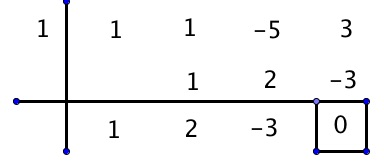# POLQ 1 | Lesson 3 | Explore (Rational Root Theorem)# Rational Root Theorem

The factoring techniques you just learned will help with factoring when the expression or function has a degree of two or lower. To factor a polynomial that has a higher degree than two, use the Rational Root Theorem. This theorem provides that the zeros of a polynomial function can be found by finding all of the possible values of  $$\Large \frac{p}{q}$$ where $$p$$ is the value of the constant term and $$q$$ is the value of the leading coefficient. This does not mean that all of the values obtained are rational roots; it means that they are possible zeroes of the function.

Use your knowledge of synthetic division with the following problems.

$f(x)=x^3+x^2-5x+3$

To find the possible zeros, factor both the constant term and the leading coefficient.

3 has factors of $$-1,\, +1,\, -3,\, +3$$

1 has factors of $$-1,\, +1$$

There are various combinations of $$\dfrac{p}{q}$$ $$-1$$ to $$+3$$. The graph below was created with Desmos. It is very helpful to see the function graphs, but please remember that there will be times when you have to find the zeros without having a graph.We can see by the graph that 1 is a zero.

Here are two ways to verify that 1 is a zero.

1) Substitute 1 for x in the function $$f(x)=x^3+x^2-5x+3$$

$$f(x)=1+1-5+3$$

$$f(1)=0$$

2) Use synthetic division.However, with synthetic division, we know that the original function can be factored to $$f(x)=(x-1)(x^2+2x-3)$$. We can use traditional factoring techniques to factor the quadratic: $$x^2+2x+3=(x-1)(x+3)$$. The zero 1 is repeated, but, $$-3$$ is another unique zero for this function. Zooming out  we can see $$-3$$ is indeed a zero. The graph below is of the same function, but zoomed out so that more of the domain and range are visible. Graph is created using Desmos.com.